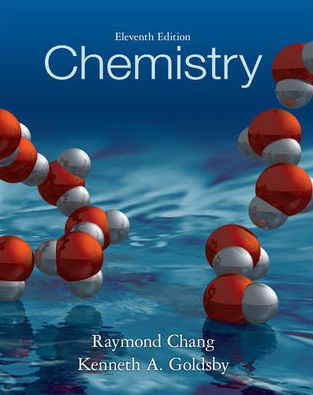×
Get Full Access to Chemistry - 11 Edition - Chapter 7 - Problem 5p
Get Full Access to Chemistry - 11 Edition - Chapter 7 - Problem 5p

×

# Planck's Quantum Theory FormulaISBN: 9780073402680 118

## Solution for problem 5P Chapter 7

Chemistry | 11th Edition

• Textbook Solutions
• 2901 Step-by-step solutions solved by professors and subject experts
• Get 24/7 help from StudySoup virtual teaching assistantsChemistry | 11th Edition

4 5 1 312 Reviews
15
2
Problem 5P

Briefly explain Planck’s quantum theory and explain what a quantum is. What are the units for Planck’s constant?

Step-by-Step Solution:

Step 1 of 2

According to Planck's quantum theory, Different atoms and molecules can emit or absorb energy in discrete quantities only.

Step 2 of 2

## Discover and learn what students are asking

Chemistry: The Central Science : Liquids and Intermolecular Forces
?The phase diagram of a hypothetical substance is (a) Estimate the norm

Statistics: Informed Decisions Using Data : The Normal Probability Distribution
?Find the z-score such that the area to the right of the z-score is 0.483.

Unlock Textbook Solution

Enter your email below to unlock your verified solution to:

Briefly explain Planck's quantum theory and explain what a